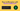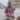# How to Memoize with React.useMemo()

Posted June 4, 2021Start discussion

From time to time React components have to perform expensive calculations. For example, given a big list of employees and a search query, the component should filter the employees’ names by the query.

In such cases, with care, you can try to improve the performance of your components using the memoization technique.

In this post, I’m going to describe how and when to use the `useMemo()` React hook.

## 1. useMemo() hook

`useMemo()` is a built-in React hook that accepts 2 arguments — a function `compute` that computes a result and the `depedencies` array:

``const memoizedResult = useMemo(compute, dependencies);``

During initial rendering, `useMemo(compute, dependencies)` invokes `compute`, memoizes the calculation result, and returns it to the component.

If during next renderings the dependencies don’t change, then `useMemo()` doesn’t invoke `compute` but returns the memoized value.

But if dependencies change during re-rendering, then `useMemo()` invokes `compute`, memoizes the new value, and returns it.

That’s the essence of `useMemo()` hook.

If your computation callback uses props or state values, then be sure to indicate these values as dependencies:

``````const memoizedResult = useMemo(() => {
return expensiveFunction(propA, propB);
}, [propA, propB]);``````

Now let’s see how `useMemo()` works in an example.

## 2. useMemo() — an example

A component `<CalculateFactorial />` calculates the factorial of a number introduced into an input field.

Here’s a possible implementation of `<CalculateFactorial />` component:

``````import { useState } from 'react';

export function CalculateFactorial() {
const [number, setNumber] = useState(1);
const [inc, setInc] = useState(0);

const factorial = factorialOf(number);
const onChange = event => {
setNumber(Number(event.target.value));
};
const onClick = () => setInc(i => i + 1);

return (
<div>
Factorial of
<input type="number" value={number} onChange={onChange} />
is {factorial}
<button onClick={onClick}>Re-render</button>
</div>
);
}

function factorialOf(n) {
console.log('factorialOf(n) called!');
return n <= 0 ? 1 : n * factorialOf(n - 1);
}``````

Try the demo.

Every time you change the input value, the factorial is calculated `factorialOf(n)` and `'factorialOf(n) called!'` is logged to console.

On the other side, each time you click Re-render button, `inc` state value is updated. Updating `inc` state value triggers `<CalculateFactorial />` re-rendering. But, as a secondary effect, during re-rendering the factorial is recalculated again — `'factorialOf(n) called!'` is logged to console.

How can you memoize the factorial calculation when the component re-renders? Welcome `useMemo()` hook!

By using `useMemo(() => factorialOf(number), [number])` instead of simple `factorialOf(number)`, React memoizes the factorial calculation.

Let’s improve `<CalculateFactorial />` and memoize the factorial calculation:

``````import { useState, useMemo } from 'react';

export function CalculateFactorial() {
const [number, setNumber] = useState(1);
const [inc, setInc] = useState(0);

const factorial = useMemo(() => factorialOf(number), [number]);
const onChange = event => {
setNumber(Number(event.target.value));
};
const onClick = () => setInc(i => i + 1);

return (
<div>
Factorial of
<input type="number" value={number} onChange={onChange} />
is {factorial}
<button onClick={onClick}>Re-render</button>
</div>
);
}

function factorialOf(n) {
console.log('factorialOf(n) called!');
return n <= 0 ? 1 : n * factorialOf(n - 1);
}``````

Try the demo.

Open the demo. Every time you change the value of the number, `'factorialOf(n) called!'` is logged to console. That’s expected.

However, if you click Re-render button, `'factorialOf(n) called!'` isn’t logged to console because `useMemo(() => factorialOf(number), [number])` returns the memoized factorial calculation. Great!

## 3. useMemo() vs useCallback()

`useCallback()`, compared to `useMemo()`, is a more specialized hook that memoizes callbacks:

``````import { useCallback } from 'react';

function MyComponent({ prop }) {
const callback = () => {
return 'Result';
};
const memoizedCallback = useCallback(callback, [prop]);
return <ChildComponent callback={memoizedCallback} />;
}``````

In the above example, `useCallback(() => {...}, [prop])` returns the same function instance as long as `prop` dependency is the same.

You can use the same way the `useMemo()` to memoize callbacks:

``````import { useMemo } from 'react';

function MyComponent({ prop }) {
const callback = () => {
return 'Result';
};
const memoizedCallback = useMemo(() => callback, [prop]);
return <ChildComponent callback={memoizedCallback} />;
}``````

## 4. Use memoization with care

While `useMemo()` can improve the performance of the component, you have to make sure to profile the component with and without the hook. Only after that make the conclusion whether memoization worth it.

When memoization is used inappropriately, it could harm the performance.

## 5. Conclusion

`useMemo(() => computation(a, b), [a, b])` is the hook that lets you memoize expensive computations. Given the same `[a, b]` dependencies, once memoized, the hook is going to return the memoized value without invoking `computation(a, b)`.

Also check the post Your Guide to React.useCallback() if you’d like to read about `useCallback()` hook.

## Quality posts into your inbox

I regularly publish posts containing:

• Important JavaScript concepts explained in simple words
• Overview of new JavaScript features
• How to use TypeScript and typing
• Software design and good coding practices